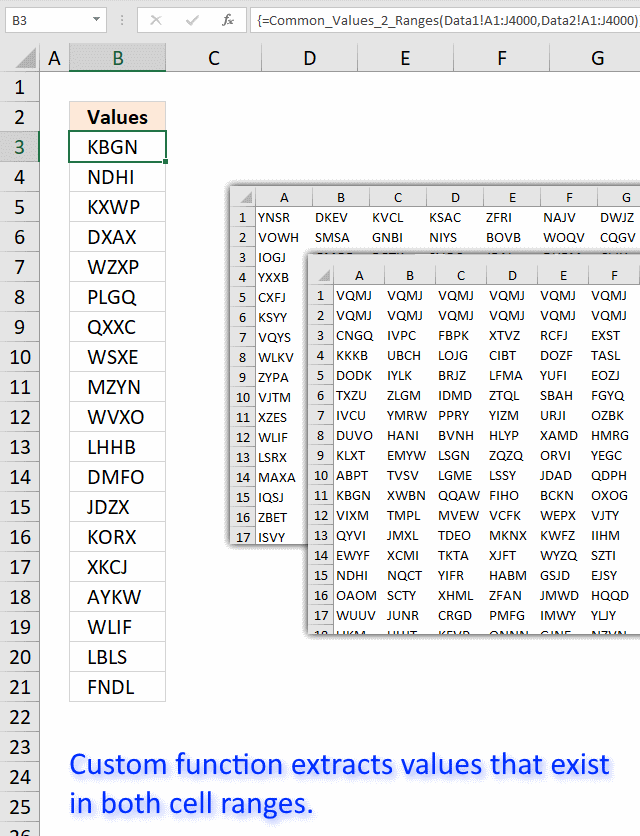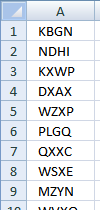Author: Oscar Cronquist Article last updated on January 07, 2019I tried the array formula in this post: Filter common values between two ranges using array formula in excel to extract common values between two cell ranges. 40000 random cell values in each cell range.

As you might have guessed, the array formula is too slow. Sheet2 contains 40000 random text strings in cell range A1:J4000, sheet3 also contains 40000 random text strings in cell range A1:J4000

This udf creates a list of common cell values between the two cell ranges:

### User defined function

```
Function Common_Values_2_Ranges(rng1 As Variant, rng2 As Variant) As Variant

Dim Value1 As Variant
Dim Value2 As Variant
Dim temp() As Variant
Dim Test As New Collection

ReDim temp(0)

rng1 = rng1.Value
rng2 = rng2.Value

On Error Resume Next
For Each Value1 In rng1
If Len(Value1) > 0 Then Test.Add Value1, CStr(Value1)
Next Value1
On Error GoTo 0

On Error Resume Next
For Each Value2 In rng2
If Len(Value2) > 0 Then Test.Add Value2, CStr(Value2)
If Err Then
temp(UBound(temp)) = Value2
ReDim Preserve temp(UBound(temp) + 1)
End If
Err = False
Test.Remove Value2
Next Value2
On Error GoTo 0

Common_Values_2_Ranges = Application.Transpose(temp)

End Function
```

### How to add the user defined function to your workbook

1. Press Alt-F11 to open visual basic editor
2. Click Module on the Insert menu
3. Copy and paste the above user defined function
4. Exit visual basic editor
5. Select sheet1
6. Select cell range A1:A5000
7. Type =Common_Values_2_ranges(Sheet2!A1:J4000, Sheet3!A1:J4000) into formula bar and press CTRL+SHIFT+ENTER
8. Make sure you save your workbook with the file extension *.xlsm so you can use the UDF the next time you open the same workbook.### Download Excel file through email

Enter your email address to receive the workbook. Note, an email will actually be sent to you.

* You will also get a weekly newsletter, unsubscribe anytime!

### Recommended blog post:

Compare two lists of data: Filter common row records in excel